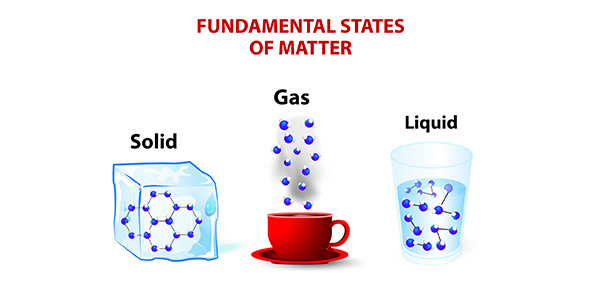# 17.0 Classification Of Matter 2

36 QuestionsSettingsRelated Topics
• 1.
Elements can be broken down into other substances by heat, light, or electricity.
• A.

True

• B.

False

• 2.
Metals
• A.

Are always magnetic

• B.

are shiny

• C.

Conduct heat and electricity

• D.

Can be pulled in wires

• 3.
Oxygen and nitrogen are
• A.

Metals

• B.

Nonmetals

• 4.
Scientists organize the elements in the
• 5.
In the periodic table, elements are organized by
• A.

Color

• B.

Weight

• C.

Properties

• D.

Shape

• 6.
Elements with similar properties are in the same column or __________________
• 7.
The smallest particle of an element that has all the properties of the element is the
• 8.
The center of an atom is the
• A.

Electron

• B.

Proton

• C.

Nucleus

• D.

Neutron

• 9.
The particle in the center of an atom that has no charge is the
• A.

Proton

• B.

Electron

• C.

Neutron

• D.

Nucleus

• 10.
The particle in the center of an atom that has a positive charge is the
• A.

Electron

• B.

Proton

• C.

Nucleus

• D.

Neutron

• 11.
The particle that is found outside of the center of the atom that has a negative charge is the
• A.

Neutron

• B.

Nucleus

• C.

Proton

• D.

Electron

• 12.
The ______________________________________ tells you the number of protons in the nucleus of the atom.
• 13.
Carbon has an atomic number of 6. How many electrons does it have?
• 14.
Two or more atoms joined together are called a __________________
• 15.
A substance made up of atoms of two or more different elements is a
• 16.
A new material developed to be used in the medical field is
• A.

Fleece

• B.

Gore-tex

• C.

Bone cement

• D.

Tang

• 17.
Good examples of mixtures are
• A.

Water

• B.

• C.

Iron filings and sand

• D.

Pyrite

• 18.
In a mixture, the atoms of the different materials are not combined so they can be easily separated.
• A.

True

• B.

False

• 19.
A special type of mixture where the particles are evenly spread out and dissolved in another material is called a
• 20.
When we describe a substance using our senses we are describing __________________ properties
• 21.
Elasticity means a substance can _______________________
• 22.
Boiling point is a
• A.

Physical property

• B.

Chemical property

• 23.
The three states of matter are
• A.

Solid

• B.

Liquid

• C.

Gas

• D.

New Jersey

• 24.
A change of state is a
• A.

Chemical change

• B.

Physical change

• 25.
A chemical property describes the way substance reacts with another substance.
• A.

True

• B.

False

• 26.
Iron filings in sand is a
• A.

Solution

• B.

Compound

• C.

Mixture

• D.

Option 4

• 27.
A substance can burn in the presence of oxygen. This is a
• A.

Physical property

• B.

Chemical property

• 28.
Iron reacts with oxygen to form______________.
• 29.
Prospectors use a __________________ property of pyrite (fool's gold) to tell it apart from gold.
• 30.
Pyrite reacts with acid to produce a ______________________.
• 31.
Digesting food is a
• A.

Physical change

• B.

Chemical change

• 32.
Baking soda combines with vinegar to produce
• A.

Hydrogen

• B.

Oxygen

• C.

Carbon dioxide

• 33.
Which physical state expands to fill up whatever container it is in?
• A.

Solid

• B.

Liquid

• C.

Gas

• 34.
Which physical state takes the shape of its container but has a definite volume?
• A.

Solid

• B.

Liquid

• C.

Gas

• 35.
Which physical sate has a definite shape and volume?
• A.

Solid

• B.

Liquid

• C.

Gas